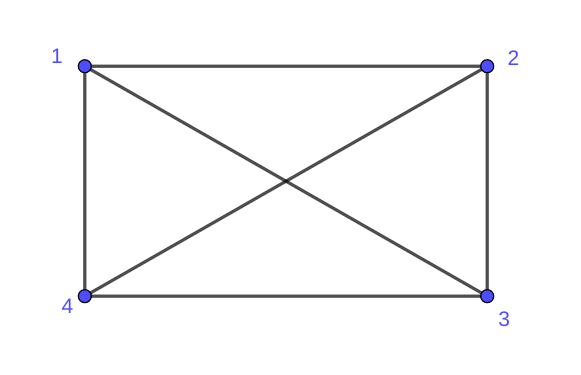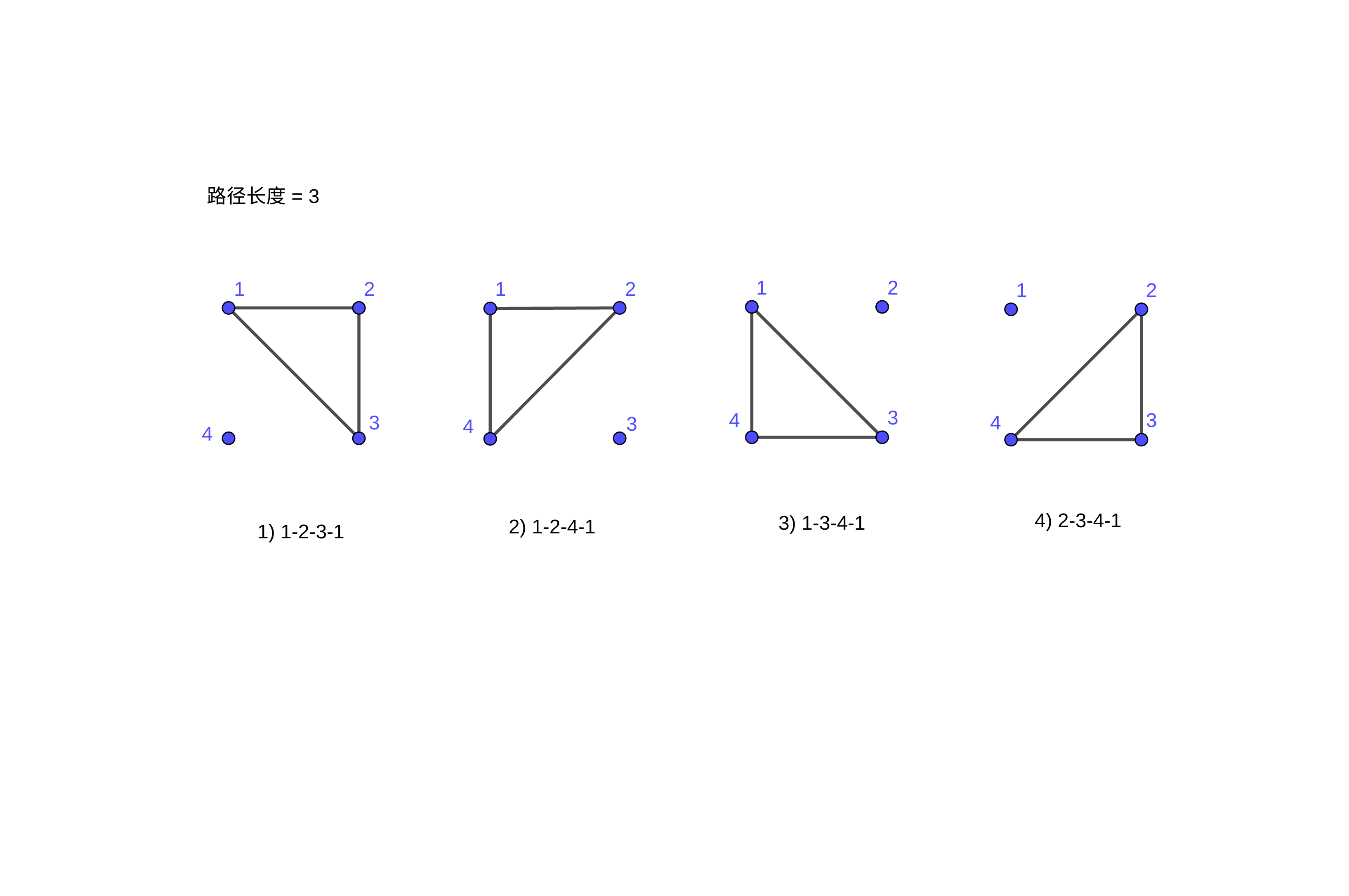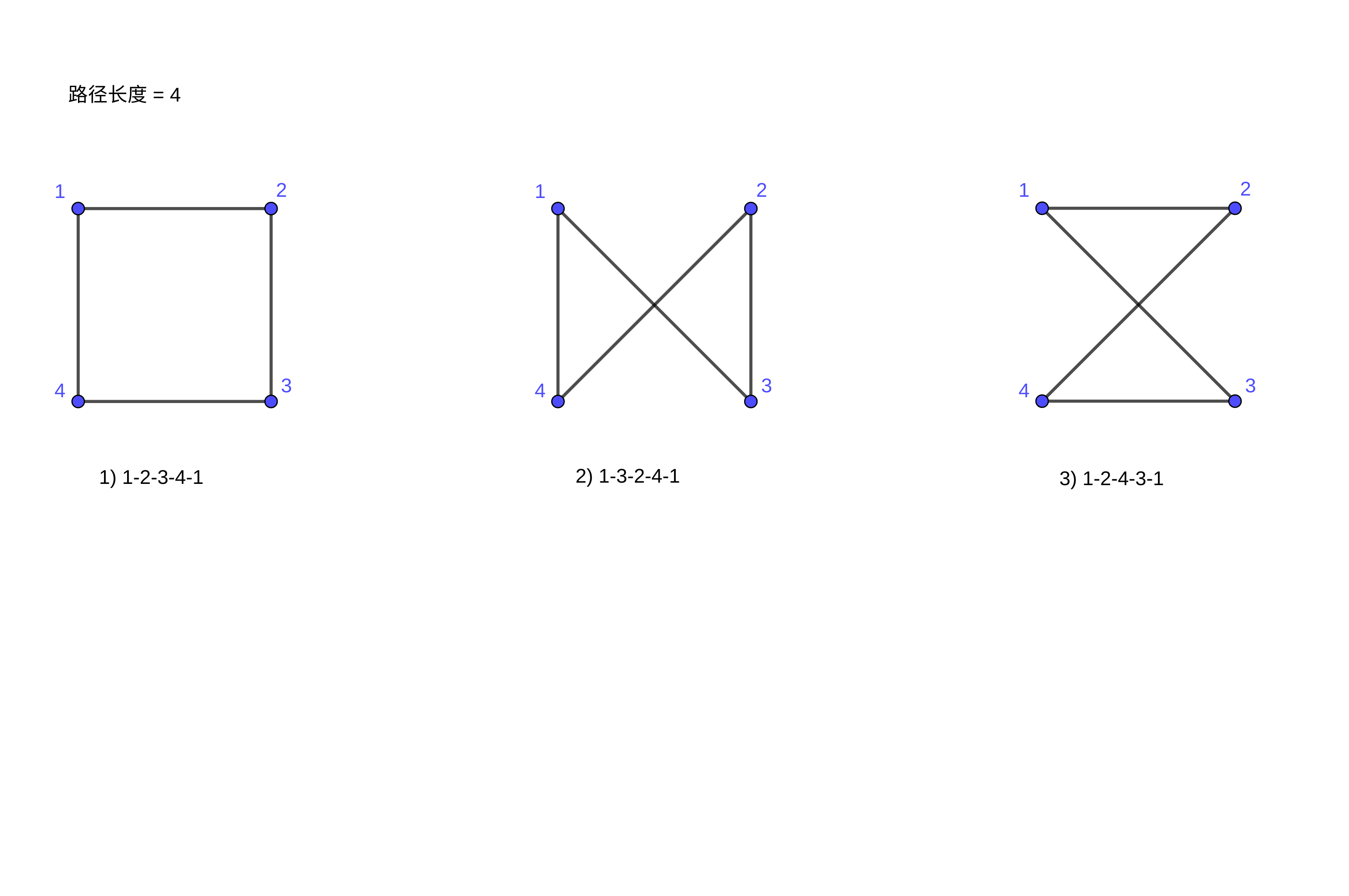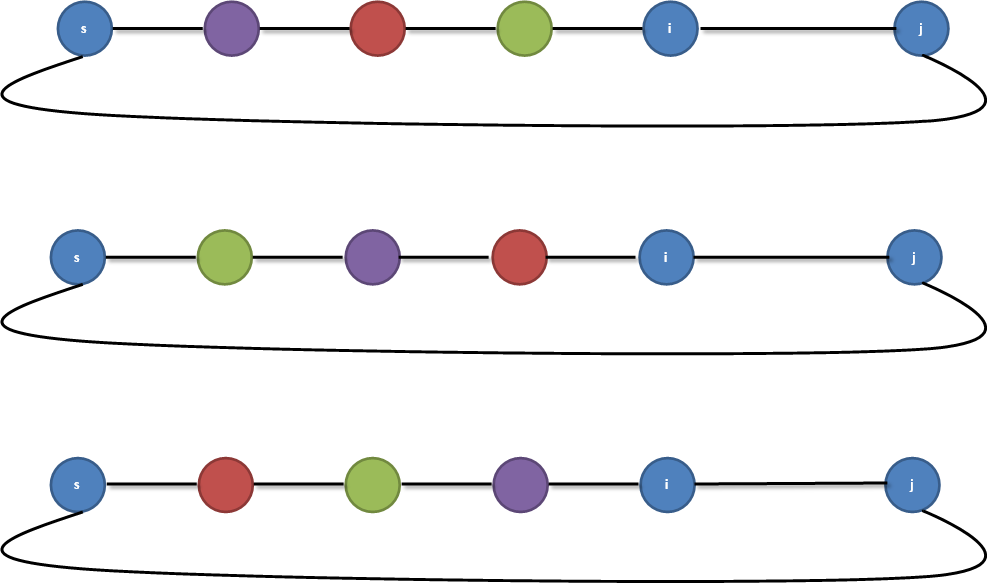# Codeforces 11D A Simple Task 统计简单无向图中简单环的个数

D. A Simple Task
time limit per test
2 seconds
memory limit per test
256 megabytes
input
standard input
output
standard output

Given a simple graph, output the number of simple cycles in it. A simple cycle is a cycle with no repeated vertices or edges.

Input

The first line of input contains two integers n and m (1 ≤ n ≤ 190 ≤ m) – respectively the number of vertices and edges of the graph. Each of the subsequent m lines contains two integers a and b, (1 ≤ a, b ≤ na ≠ b) indicating that vertices a and b are connected by an undirected edge. There is no more than one edge connecting any pair of vertices.

Output

Output the number of cycles in the given graph.

Examples
input
Copy
4 6
1 2
1 3
1 4
2 3
2 4
3 4

output
Copy
7

Note

The example graph is a clique and contains four cycles of length 3 and three cycles of length 4.dp[SET][j]=∑(iSET,ij)dp[SET][i]

#include<bits/stdc++.h>
using namespace std;
typedef long long ll;
const int maxn = 20;
int maze[maxn][maxn];
// dp[s][i] : 表示ｓ状态下以　s 状态的最小顶点和顶点i构成的简单环　(这样仍然会重复计算2次)
ll dp[1<<maxn][maxn];
int n,m;
int main()
{
while(~scanf("%d%d",&n,&m))
{
memset(dp,0,sizeof(dp));
memset(maze,0,sizeof(maze));
for(int i=0,u,v;i<m;i++) {
scanf("%d%d",&u,&v);
u--,v--;
maze[u][v] = maze[v][u] = 1;
}
ll ans = 0;
for(int i=0;i<n;i++) dp[1<<i][i] = 1;
for(int s=1;s<(1<<n);s++) {
int pre = log2(s & -s);
for(int i=pre;i<n;i++) if(dp[s][i]){ /// 枚举结尾的顶点
for(int j = pre;j<n;j++) if(maze[i][j]){ /// 枚举接下来要连接的顶点
if(s & (1<<j)) { ///　节点j在当前状态中
if (((1<<i)|(1<<j))==s) continue; /// 排除两个节点成环的情况
if(j == pre) ans += dp[s][i];
}
else { /// 节点j不在当前状态中
dp[s|(1<<j)][j] += dp[s][i];
}
}
}
}
printf("%lld\n",ans/2);
}
return 0;
}